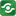Logarithmic and Trigonometric Functions Calculator@import url(http://www.google.com/cse/api/branding.css);CSGNetwork Custom SearchCSGNetwork Snippets Calculators Top Free Apps Home Back

Right click this window and select "view source" in order to copy the source for this script.

 Logarithmic and Trigonometric Functions Calculator This calculator will calculate logarithms, trigonometric functions, and the logarithms of trigonometric functions. Enter the value you want to apply the function to in the field on the right. To calculate the logarithm of the value, check the logarithm box. To calculate the trigonometric function on the value, select the desired function from the list. To calculate the logarithm of a trigonometric function applied to the value, check the logarithm box and choose a trigonometric function.
Check to compute the logarithm:
 log10
Choose a trigonometric function (or none):
 none sine cosine tangent cotangent secant cosecant
Enter a value:
 (Enter all angles in decimal degrees)
 Result:

View the source of this Logarithmic and Trigonometric Functions Calculator script...